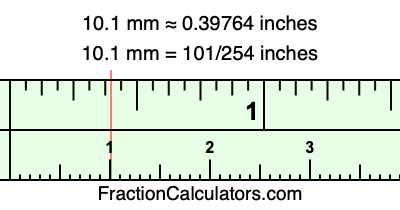10.1 mm in inchesHere is how to convert 10.1 mm to inches. We will give you the fractional answer, the decimal answer, and illustrate the answer on our tape measure.

One millimeter is equal to 5/127 inches, and 10.1 as a fraction is 101/10. Therefore, to get the fractional answer to 10.1 mm in inches, we multiply 101/10 by 5/127 and simplify if necessary.

cm × 5/127 = inches
101/10 × 5/127 = inches
101/10 × 5/127 = 101/254
101/10 mm = 101/254 inches
10.1 mm = 101/254 inches

As promised above, we also have the answer to 10.1 mm in inches in decimal form. Although the fractional answer above is exact, the decimal answer may be rounded if necessary:

101/254 ≈ 0.397637795275591
101/254 ≈ 0.39764
10.1 mm ≈ 0.39764 inches

Our image below shows 10.1 mm on a tape measure. The top row of the tape measure is inches, and each mark on the bottom row is 1 millimeter (10 millimeter marks equal 1 centimeter).As you can see, we drew a red line where 10.1 mm and 0.39764 inches meet on the measuring tape.

Millimeters to Inches Calculator
Here you can convert another length in millimeters to inches.

Convert  mm to inches.

What is 10.2 mm in inches?
Here is another inches to millimeters calculation that we have done for you.